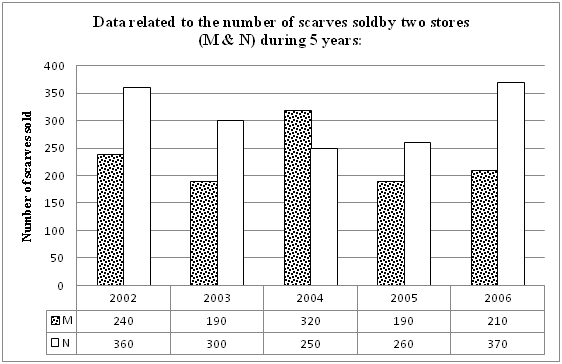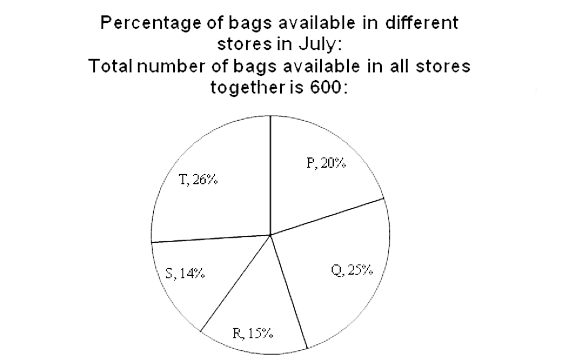# Sample Aptitude Questions of E & Y

DIRECTIONS for questions 1to 5: Refer to the graph and answer the given question:1. What is the difference between the total number of scarves sold by store M in 2003 and 2004 together and total number of scarves sold by store N in 2005 and 2006 together?
1. 160
2. 100
3. 140
4. 150
5. 120
Total Number of scarves sold store M in 2003 and 2004= 190+320= 510
Total Number of scarves sold store M in 2005 and 2006=260+370= 630
Difference between the total Number of scarves sold store M in 2003 & 2004 and
Total Number of scarves sold store N in 2005 and 2006 is = 630 – 510 = 120.
1. Number of scarves sold by store M decreased by what percent from 2004 to 2005?
1. 40 5/8
2. 45 3/8
3. 42 3/8
4. 30 3/8
5. 35 5/8
Percentage decrease in the number of scarves sold by score M =
320-190/320 * 100 = 130/320 * 100 = 40 5/8%
2. If the respective ratio between total number of scarves sold by stores M and N together in 2002 and that in 2009 is 15 : 11, what is the total number of scarves sold by stores M and N together in 2009?
1. 430
2. 450
3. 420
4. 460
5. 440
Ratio between total number scarves sold by stores M & N together in 2002 and that in 2009 is 15:11.
Total number scarves sold by stores M & N together in 2002 = 240+360 = 600
According to question,= 600/x = 15/11 Or, x = 440
Total number scarves sold by stores M & N together in 2009 = 440.
3. If the total number of scarves sold by stores M and N together in 2008 is 10% more than that in 2006, what is the total number of scarves sold by stores M and N together in 2008?
1. 638
2. 406
3. 414
4. 396
5. 408
Total number of scarves sold by stores M & N in 2006 = 210+370 = 580
Total number of scarves sold by stores M & N in 2008 = 580×110/100 = 638
4. What is the average number of scarves sold by store N in 2003, 2004 and 2005?
1. 260
2. 270
3. 290
4. 250
5. 290
Average number of scarves sold store N in 2003, 2004 & 2005 = 300+250+260 /3 = 2705. What is the central angle corresponding to number of bags available in store T? (In degrees)
1. 91.2
2. 95.6
3. 93.6
4. 94.2
5. 92.5
Central angle corresponding to number of bags available in store T = 26/100*360= 93.6 Deg
6. What is the difference between the average number of bags available in stores P and R together and the average number of bags available in stores S and T together?
1. 12
2. 22
3. 15
4. 18
5. 20
Average percentage of bags available in stores P and R together = 35%/2 = 17.5%
Average percentage of bags available in stores S and T together = 40%/2 = 20%
Difference between Average Number of bags available in stores P and R together and
Average Number of bags available in stores S and T together = (20% - 17.5%) of 600 = 15
7. The respective ratio between number of bags available in store P in August and that available in the same store in July was 5: 4. How many bags were available in store P in August as compared to July?
1. 150
2. 90
3. 24
4. 60
5. 45
Number of bags available in P store in July = 20% of 600 = 120.
Now, since we have
Number of bags available in store P in August : Number of bags available in P store in July = 5:4
=> P : 120 = 5 : 4
Thus, number of bags available in Store P (In August) = (5/4)x120 = 150
Universities Total Number of faculty members Percentage of Assistant professors Number of Associate professors
J 250 60 75
K 180 75 24
L 150 80 16
M 100 63 21
8. What is the difference between the total number of Associate Professors in Universities J and M together and the total number of Professors in the same universities together?
1. 54
2. 55
3. 68
4. 58
5. 53
Universities Total Number of faculty members Percentage of Assistant professors Number of Associate professors
J 250 60 75
K 180 75 24
L 150 80 16
M 100 63 21

Total number of Associate professor in universities J and M together = 75+21= 96
Total number of professor in universities J and M together = 25+16= 41
Difference between the Total number of Associate professor in universities J and M together and Total number of professor in universities J and M together= 96- 41= 55
9. In University M, 8/21 of the Assistant Professors are males and in University L, 3/5 of the Assistant Professors are males. What is the respective ratio between male assistant Professors in University M and that in University L?
1. 2:5
2. 1:3
3. 3:5
4. 2:7